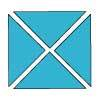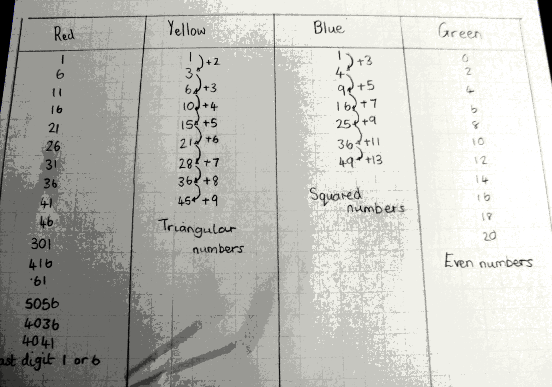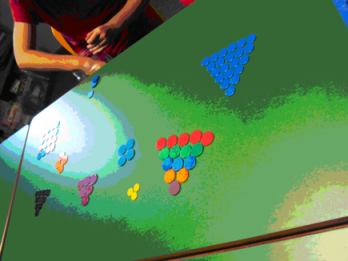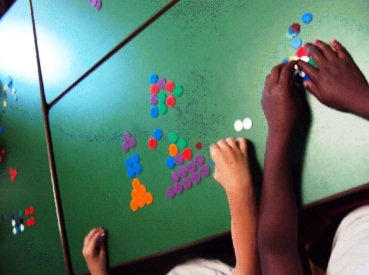#### You may also like### Chocolate

There are three tables in a room with blocks of chocolate on each. Where would be the best place for each child in the class to sit if they came in one at a time?### Four Triangles Puzzle

Cut four triangles from a square as shown in the picture. How many different shapes can you make by fitting the four triangles back together?### Cut it Out

Can you dissect an equilateral triangle into 6 smaller ones? What number of smaller equilateral triangles is it NOT possible to dissect a larger equilateral triangle into?

# Light the Lights Again

##### Age 7 to 11 Challenge Level:

Many of you arrived at the same correct conclusion that $36$ is the smallest number which lights up all the lights.

Some used trial and improvement, testing out all the numbers from $1$ until they reached $36$. This is one way to answer the question, but what we'd really like to know is: what are the rules behind the lights?

Students in Mrs Keary's Maths Group of Bushloe High School summed this point up well: We worked on this problem for a whole lesson and only just found all of the reasons right at the end, though we did find $36$ quite quickly!

Class 4 of Grampian Primary School said:

The first pattern we spotted was that all the even numbers would light the 'Green' light. We experimented with larger numbers to check that this still worked. We agreed that 'any multiple of $2$ would light the Green light'.

The second pattern we spotted was that the 'Red' light would light up when you entered a number that ended in $6$ or $1$. We tested this with four digit numbers and it still worked. We agreed that 'any number ending in $1$ or $6$ would light up the Red light'.

Year 6 of the Croft School had a good approach which made it easy to identify the rule for the Red light:

We started off using guess and check and wrote down what lights lit up. We found that $36$ lit all the lights but we did not know why. We decided to try the lights from $1$, and to colour in a hundred square to look for patterns for each colour. We noticed the red squares formed columns downwards, like the $5$ times table does, but they are not $5$ times table, you start with $1$ and add $5$ each time.

Class 4 continued:

The Yellow and Blue lights were much more difficult to spot. We started to look at the number sequences and looked at what you had to do to one number to get to the next number. We spotted a pattern and could then say what the next number would be. We tested these in the problem. Here is a copy of our recordings.Many of you correctly identified the numbers in the Yellow column as the Triangular numbers and the numbers in the Blue column as the Square numbers.

Finlay and Ben of St Andrew's School wrote down all the formulae which lit up all the lights:

Green   = Even number            = $2n$
Yellow  = Triangular numbers = $n(n+1)\over 2$
Blue      = Square number         = $n^2$
Red       = Every five number   = $5n+1$

Here are some more pictures sent into us by Class 4 of Grampian Primary School investigating triangular numbers and square numbers with counters.